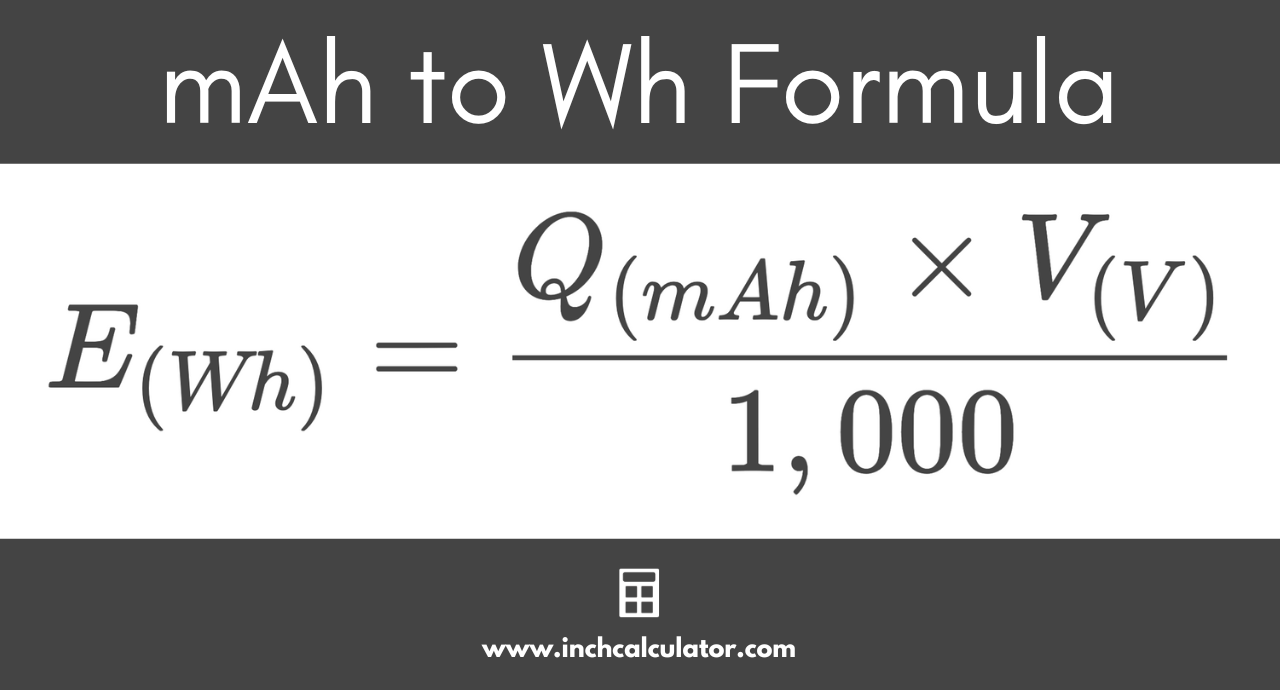# Milliamp-Hours (mAh) to Watt-Hours (Wh) Conversion Calculator

Convert milliamp-hours to watt-hours by entering the amount of charge in mAh and the battery terminal voltage below.

mAh
V

## Watt-Hours Results:

Wh
Learn how we calculated this below

## How to Convert Milliamp-Hours to Watt-Hours

Units like milliampere-hours (mAh) and watt-hours (Wh) are commonly used to describe the battery capacity. You might need to convert mAh to Wh, so follow along, and we’ll show you how.

While it is impossible to measure the internal characteristics of a battery because it is a closed system, we can use the parameters observable at the battery terminals to estimate the internal measures like stored charge and total energy capacity.

The milliampere-hour capacity of a battery describes the duration for which a battery can supply one milliampere of current and the maximum amount of current it can supply for an hour.

For example, a 2 mAh of capacity means that the battery can supply a load of 2 milliamperes for one hour before losing all its charge, or it can indicate that the battery can supply a 1 milliampere current to a load for 2 hours. The electric charge for smaller batteries is generally measured in milliamp-hours, abbreviated mAh or mA·h.

The watt-hour capacity of a battery, expressed as Wh or W·h, is a measure of the total electrical power the battery can supply for an hour or the total duration for which it can supply a specific load. It is a measure of the amount of energy being supplied to the load.

### mAh to Wh Conversion Formula

The conversion from electric charge to energy requires the voltage and can be done using the formula below.

E(Wh) = Q(mAh) × V(V) / 1,000

Thus, the energy E in watt-hours is equal to the charge Q in milliamp-hours times the voltage V, divided by 1,000.By reversing this formula, you can also convert Wh to mAh.

For example, let’s convert the charge used by an average C battery with a charge of 8,000 mAh at 1.5 V to Wh.

E(Wh) = 8,000 mAh × 1.5 V / 1,000
E(Wh) = 12,000 mWh / 1,000
E(Wh) = 12 Wh

So, an 8,000 milliamp charge at 1.5 volts is equal to 12 watt-hours of energy.

## Milliamp-Hours to Watt-Hours Conversion Chart

Table showing milliamp-hour converted to watt hours for common battery sizes
Battery Size Capacity (mAh) Energy (Wh) Voltage (V)
AA 2,700 mAh 4.05 Wh 1.5 V
AAA 1,200 mAh 1.8 Wh 1.5 V
AAAA 625 mAh 0.9375 Wh 1.5 V
C 8,000 mAh 12 Wh 1.5 V
D 12,000 mAh 18 Wh 1.5 V
9V 565 mAh 5.085 Wh 9 V

Try converting amp-hours to kilowatt-hours.